# Solving the Jane Street Puzzle of April 2020; Backtrack with OCaml

May 01, 2020Wassily Kandinsky, Composition VIII, 1923

Every month, Jane Street Capital post a puzzle on their website. This was the puzzle for April 2020.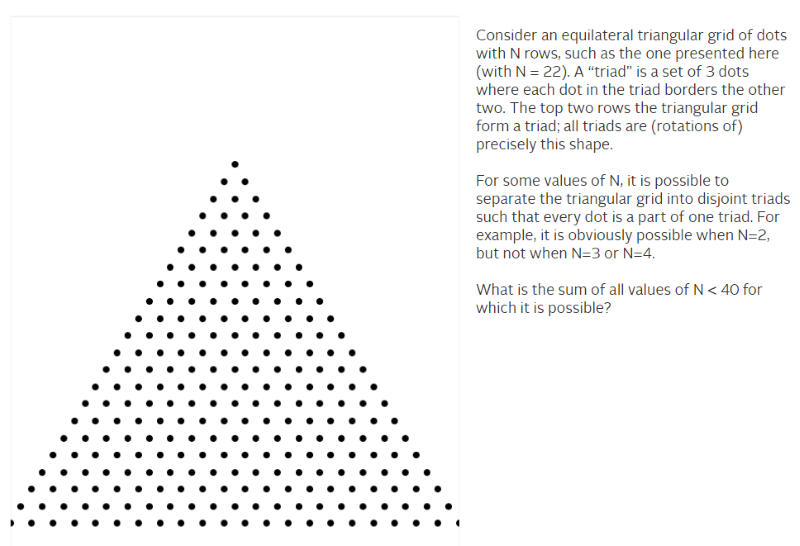## Approach

I started to solve the puzzle by hand in order to understand the problem space better and to find patterns. Solving the triangular grid by hand is quite easy for lower value of N. Example for N=9.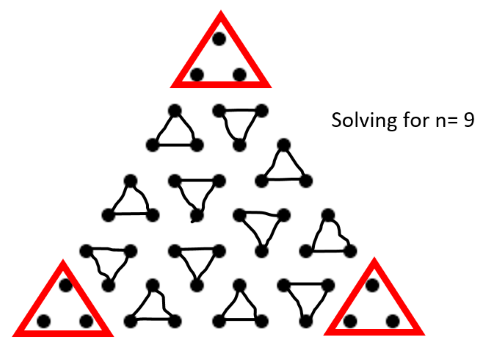Some observations:

• I was placing the triads along the sides first (vs in the middle). Thus using a constraint of the sides to eliminate some of the infeasible options early on
• When I was unable to place a triad, I would go back and try some other option; backtracking
• The three corners (marked in red above) can be ignored from the solution as they always have the same triad placement
• Every triad can only be placed in one of two ways. Either pointing up like a pyramid or pointing down

A solution is only possible if the number of dots is multiple of 3. This reduced the problem space by a third.

``````Number of dots      = (N + 1) * N / 2
Number of triads    = dots / 3
= (N + 1) * N / 6

--       ----  -----------
1	   1	0.333333333
2	   3	1
3	   6	2
4	  10	3.333333333   <--- infeasible, as the number of dots is not divisible by 3
5	  15	5
6	  21	7
7	  28	9.333333333
8	  36	12
9	  45	15
10	  55	18.33333333
11	  66	22
.
.
37	 703	234.3333333
38	 741	247
39	 780	260
``````

## Backtracking my way into the solution

At this point my thinking was to automate the manual (by hand) method by creating a program based on the depth-first search (DFS) backtracking algorithm. Backtracking can be used to solve many well known problems such as suduko  and the eight queens puzzle.

A concern was that the run-time might be very long for high values of N. Worse case the run-time is polynomial - O(n^k), with k somewhere between 2-4. However with the known constraints such as the the sides and corners, it might not be so bad.

The program I created was called `triad.exe`. More about the program a bit later.

Running `triad.exe`

``````  N      dots    triads  solve
--   -------   -------  -----
1         1       0.3      -
2         3       1.0      1
3         6       2.0      -
4        10       3.3      -
5        15       5.0      -
6        21       7.0      -
7        28       9.3      -
8        36      12.0      -
9        45      15.0      1   <----- solve = 1 means that a valid solution exists
10        55      18.3      -
11        66      22.0      1
12        78      26.0      1
13        91      30.3      -
14       105      35.0      1
15       120      40.0      -
16       136      45.3      -
17       153      51.0      -
18       171      57.0      -
19       190      63.3      -
20       210      70.0      -
21       231      77.0      1
22       253      84.3      -
23       276      92.0      1
24       300     100.0      1
25       325     108.3      -
26       351     117.0      1
^C
``````

It took less than a second to solve up until N=26. For N=27 – the calculation took way too long, so I stopped it. However, I spotted a pattern. It can be solved for N = 9, 11, 12 & 14 and then again for 21, 23, 24 & 26. It appears that this pattern might repeat itself for every 12th value of N. With this in mind I tested it for N=33 onwards (which is N=21 +12)

`triad.exe 33`

``````  N      dots    triads  solve
--   -------   -------  -----
33       561     187.0      1
34       595     198.3      -
35       630     210.0      1
36       666     222.0      1
37       703     234.3      -
38       741     247.0      1
^C
``````

Bingo! What about from N=45, which is +12 from N=33?

`triad.exe 45`

``````  N      dots    triads  solve
--   -------   -------  -----
45      1035     345.0      1
46      1081     360.3      -
47      1128     376.0      1
48      1176     392.0      1
49      1225     408.3      -
50      1275     425.0      1
^C
``````

Same story. Why this pattern and what about the values inbetween these? I was not 100% sure at this stage. But, with the clock ticking and some confidence I submitted my answer which got my name on the Correct Submissions list.

284, which is 2 + 9 + 11 + 12 + 14 + 21 + 23 + 24 + 26 + 33 + 35 + 36 + 38

## Patterns

So why does the pattern repeat and what about the other values?

We already know that N=9 is valid - as it was solved by hand. By simply adding rows of triads, we can extend the valid solutions to N=11 and also for N=12 and N=14.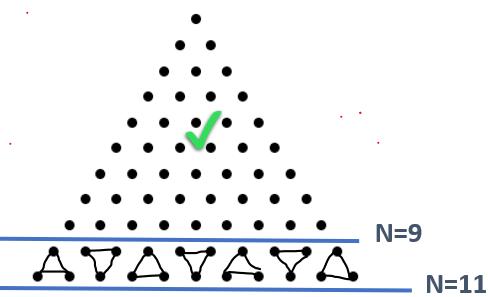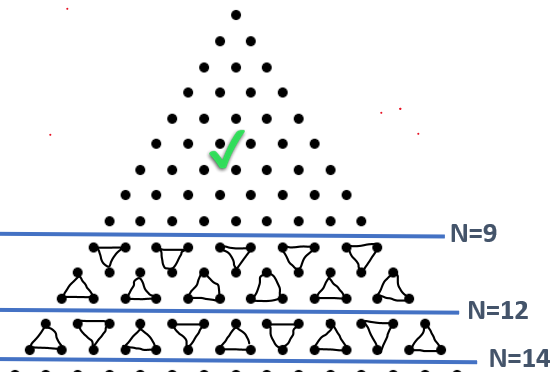Similarly, the N=21 triangle can be build up from other triangles that are all valid solutions. Starting with N=9 and adding triangles where N=9 and N=12. As per initial obervations the corner triads can be ignored if need be. This same pattern repeats every 12th row.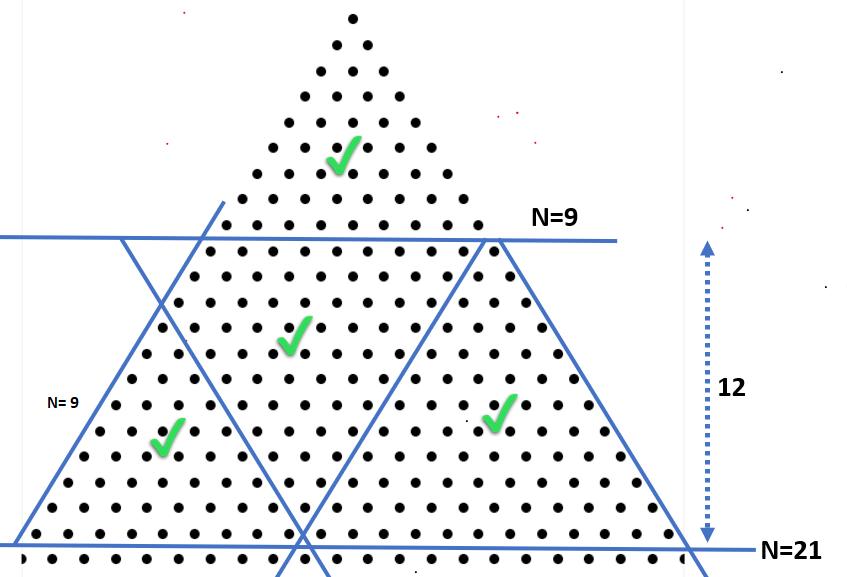So the backtracking program was probably not required, but it was definitely a good way to validate the solution.

## Using OCaml

I mostly use Python for this kind of puzzles. However, similar to my solution to the February 2020 Jane Street Puzzle, I decided to rather use OCaml. This was mainly to improve my OCaml skills but also for performance.

An `Array` is used to model the triangular grid which is created with the `create_grid` function in the code.

``````   Grid Coordinates

|  1  2  3  4
-+-----------------> x-value
|
1  !  0  1  1  1           Every 0 is a dot in the triangular grid
|                       Sample here is where N=4
2  |  0  0  1  1
|                       If grid value is:
3  |  0  0  0  1           0 -> empty, available to place a triad
|                       1 -> occupied / unavailable
4  |  0  0  0  0
|
y-value
``````

Triads can be placed in the grid pointing `Up` (like a pyramid) or `Down` (inverted pyramid). This is done by the `change_grid` function. The `X` in diagram below refer to the dot being evaluated. The coordinate of `X` is determined by the `next_dot` function. A triad placement `is_valid` if all three points of the triad are empty (0).

``````     Up         Down

X         X 0
0 0         0
``````

See below for the the full program. I used the OCaml for Windows  compiler. If you don’t have an OCaml compiler, you can run the code by using one of the online notebooks such as https://sketch.sh/new/ml.

``````(* triangle is marked with 0's, borders with 1's *)
let create_grid size =
let grid = Array.make_matrix (size + 2) (size + 2) 1 in
for y = 1 to size  do
for x = 1 to size do
if x <= y then grid.(x).(y) <- 0
done
done;
grid

(* find next open space for triad placement *)
let next_dot grid =
let x' = ref 0 in
let y' = ref 0 in
let size = Array.length grid - 2 in
try
for x = 1 to size do
for y = 1 to size do
if grid.(x).(y) = 0 then
begin
y' := y;
x' := x;
raise Exit
end
done
done;
None
with Exit -> Some (!x', !y')

type triad = Up | Down

let is_valid x y placement grid =
assert (grid.(x).(y) = 0);
match placement with
| Up -> grid.(x).(y+1) = 0 && grid.(x+1).(y+1) = 0
| Down -> grid.(x+1).(y) = 0 && grid.(x+1).(y+1) = 0

(* place or remove the triad *)
let change_grid x y placement grid value =
match placement with
| Up -> grid.(x).(y) <- value;
grid.(x).(y+1) <- value;
grid.(x+1).(y+1) <- value
| Down -> grid.(x).(y) <- value;
grid.(x+1).(y) <- value;
grid.(x+1).(y+1) <- value

let rec solve grid =
let dot = next_dot grid in
match dot with
| None -> true
| Some (x, y) ->
let check placement =
if is_valid x y placement grid then
begin
change_grid x y placement grid 1;
if solve grid then true else
begin
change_grid x y placement grid 0;
false
end
end
else false in
List.exists (fun z -> check z) [Up; Down]

Format.printf "  N      dots    triads  solve\n%!";
Format.printf " --   -------   -------  -----\n%!"

let () =
for n = 1 to 26 do
let dots = (n + 1) * n / 2 in
let triads = float_of_int dots /. 3. in
let result  =
if (dots mod 3 = 0) && (create_grid n |> solve)
then "1" else "-" in
Format.printf "%3i %9i %9.1f %6s \n%!" n dots triads result
done
``````

In my case I set the start value with a command line argument and used the `Unix.gettimeofday()` function to measure execution time. This was excluded from the code above as the `Sys` and `Unix` module will only work on local installations of OCaml (including Windows).

``````let () =
let i = try int_of_string Sys.argv.(1) |> max 1 with _ -> 1 in
let t = Unix.gettimeofday () in
for n = i to 99 do
let dots = (n + 1) * n / 2 in
let triads = float_of_int dots /. 3. in
let result  =
if (dots mod 3 = 0) && (create_grid n |> solve)
then "1" else "-" in
Format.printf "%3i %9i %9.1f %6s %10.1f \n%!"
n dots triads result (Unix.gettimeofday () -. t)
done
``````

Commands used to test, compile or profile the code.

``````# testing

# final version

# Profiling
``````

Although the program above assisted me to find the correct answer, it was very inefficient. Here are some of the obvious changes that can be made:

• Don’t calculate the next dot by scanning all the items again. Rather have a pre-determined path and follow that
• Use a search path for dots that start on the edges of the grid. That way many infeasible options can be ruled out early on
• Pre-fill the corners with triads, as these placements are known

A comment on style. I used ‘Exceptions’ in my code - see the use of `raise` in the `next_dot` function. This approach is seen as not good style. As per Jane Street Style Guide:

Although raising is fine, one should not write code that depends on which exception is raised.

Another possible improvement area is not to use an `Array` to keep track of the solution space.

This was a lot of fun. Thanks to Jane Street Capital for posting the puzzle. If you can suggest changes to the code or have also solved this puzzle, let me know.

 Jane Street - Puzzle Archive.
https://www.janestreet.com/puzzles/archive/. Retrieved: 2020-04-03

 Source code for this post on Github.
https://github.com/whoek/janestreet-puzzles/tree/master/2020-04. Retrieved: 2020-05-01

 OCaml for Windows - Graphical Installer
https://fdopen.github.io/opam-repository-mingw/installation/. Retrieved 2020-05-01

 Backtracking Iterators, Jean-Christophe Filliatre
https://www.lri.fr/~filliatr/publis/enum2.pdf. Retrieved: 2020-04-10

 Functional Programming - Lecture 6: Folding and Backtracking, by Łukasz Stafiniak

 OCaml - Sudoku par backtrack
https://openclassrooms.com/forum/sujet/ocaml-sudoku-par-backtrack

## Related Posts

Solving the Jane Street puzzle of December 2022

Why I created Scrumdog - a program to download Jira Issues to a local database

Jane Street puzzle Feb 2021 SOLVED! OCaml to the rescue

Solving the Jane Street puzzle of Dec 2020 - Backtracking with OCaml

Automate your Jira reporting with Python and Excel

Solving the Jane Street Puzzle of June 2020; Circle Time